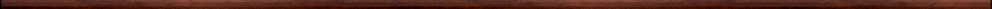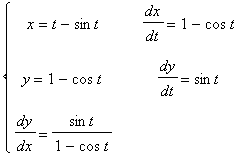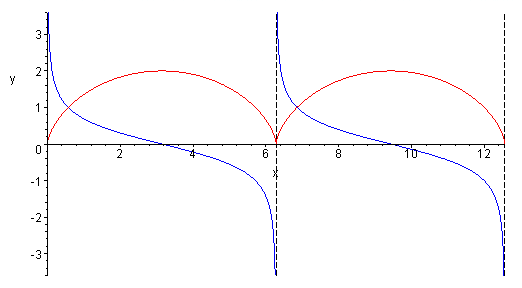More Maple worksheets on differentiationBasic calculus topics:

They are all compatible with Classic Worksheet Maple 10.CYCLOIDImplicit functions - implctfn.mws

• Graphs of implicit functions

Implicit differentiation - impdiff.mws

• The method of implicit differentiation.
• Implicit differentiation in action.
• Some standard expressions which occur when differentiating implicitly.
• Implicit differentiation using diff.
• Tangent lines to general curves defined by an equation involving two variables.
• Existence of derivatives of implicit functions.

Parametric curves - param.mws

• Graphs of implicit functions.
• The gradient of a parameterized curve.
• Determining the gradient of some parameterized curves.
• The cycloid.
• The speed of a point moving along a curve.
• The speed of a point on the rim of rolling wheel.

Some interesting curves - curves.mws

• Lemniscate.
• Dumb-bell.
• Cissoid.
• Bifoliate.
• Trefoil.
• Maltese Cross.

Derivatives of trig functions - difftrig1.mws

• The derivatives of the sine and cosine functions.
• A more formal treatment of the two limits needed

Derivatives of trig functions II - difftrig2.mws

• Graphical illustration of the derivatives of the sine and cosine functions.
• Derivatives of other functions defined using trig functions.

Derivatives of trig functions III - difftrig3.mws

• Derivatives of tan, sec, cosec, cot.

Exponential and logarithm functions - explog.mws

• Logarithm functions.
• The derivative of the general logarithm function.
• The exponential function.
• Some graphs involving the exponential function.